#2017 iT 邦幫忙鐵人賽
DAY 23
1
Big Data

## Day23 R語言機器學習之簡單迴歸分析

• 簡單線性迴歸 Simple Linear Regression
• 羅吉斯迴歸  Logistic Regression
• 複迴歸 Multiple Regression

• 如果依變數Y也是一個數值，就可以是著套線性迴歸。
• 如果依變數Y是二元型別，這時羅吉斯迴歸分析是很常使用的演算法。

``````Y = a + bX + e
``````

a是常數
b是相關係數
e是誤差值，希望是服從常態分佈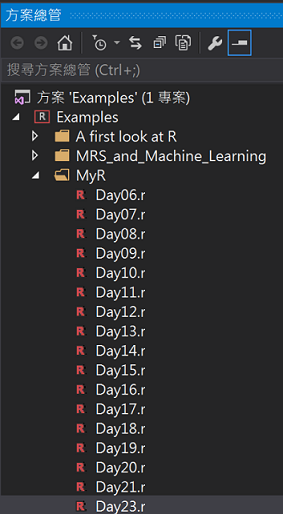## 什麼時候可以用迴歸分析?

Speed 速度
dist 煞車距離

#先畫散佈圖觀察

``````ggplot(cars, aes(x = speed, y = dist)) + geom_point(shape = 10, size = 5)
``````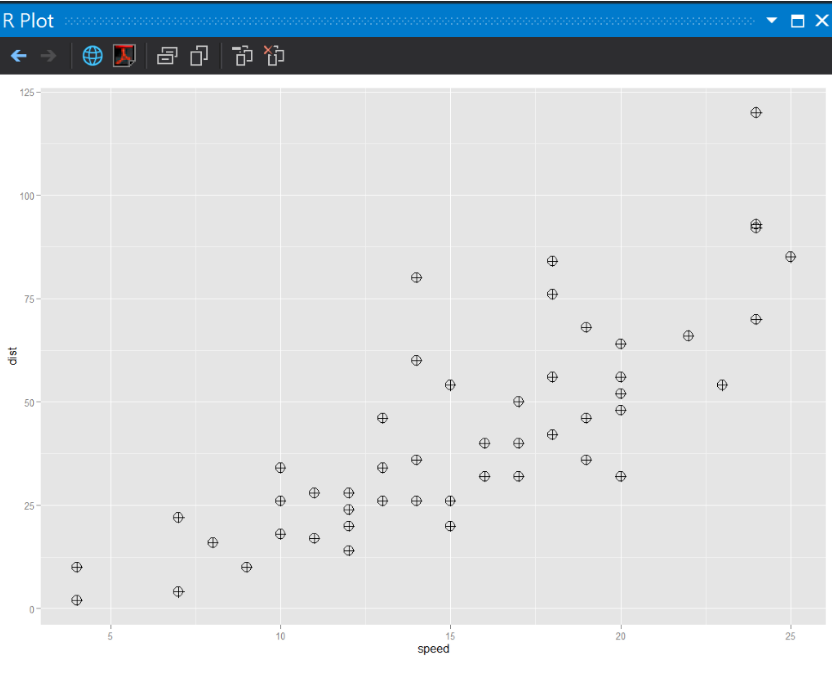## 訓練模型

``````#lm(y~x)
carsLM <- lm(dist ~ speed, data = cars)
#散佈圖 加上模型預測區域
ggplot(cars, aes(x = speed, y = dist)) + geom_point(shape = 10, size = 5) +
geom_smooth(method = lm) + labs(x = "速度", y = "煞車距離")
``````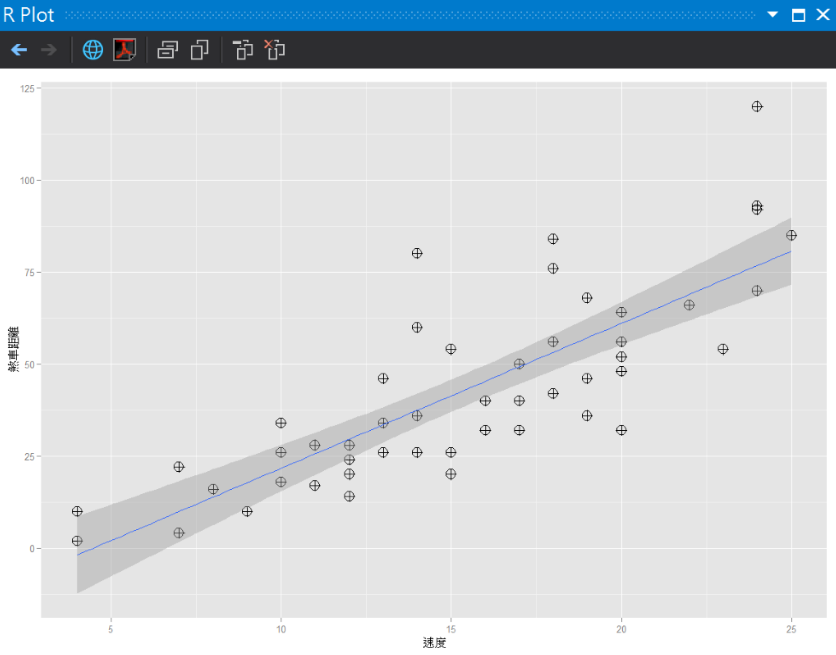## 方程式係數取得

``````summary(carsLM)
```````R-squared`是簡單評估迴歸模型預測準度的數值，圖中為0.6438，越接近1，解釋力越強大。

Y = a + bX + e

Y= -17.5791 + 3.9324 * 20
=  61.0689

## 利用預測函數取得結果

``````#(4)預測
new <- data.frame(speed = 20)
result <- predict(carsLM, newdata = new)
result
````````````#(5)把預測座標放到圖上
ggplot(cars, aes(x = speed, y = dist)) + geom_point(shape = 10, size = 5) +
geom_point(x = new\$speed, y = result, size = 10, shape = 17, color = "red") +
geom_smooth(method = lm) + labs(x = "速度", y = "煞車距離")
``````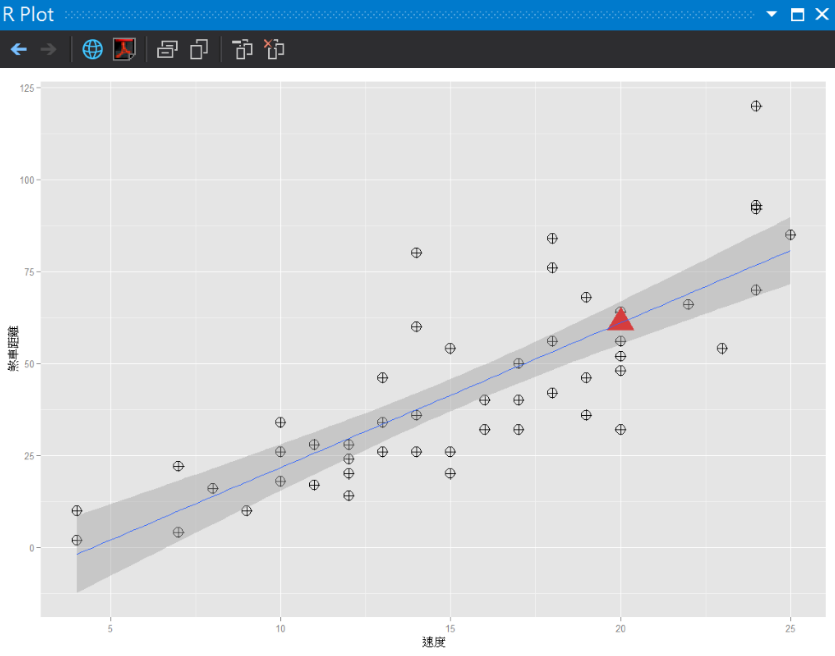``````#來個card2從英制轉換為公制
cars2 <- cars
#一英哩 = 1.6公里
cars2\$speedByMetric <- cars\$speed * 1.6094
#一英尺 = 0.3048公尺
cars2\$distByMetric <- cars\$dist * 0.3048
ggplot(cars2, aes(x = speedByMetric, y = distByMetric)) + geom_point(shape = 10, size = 5)
``````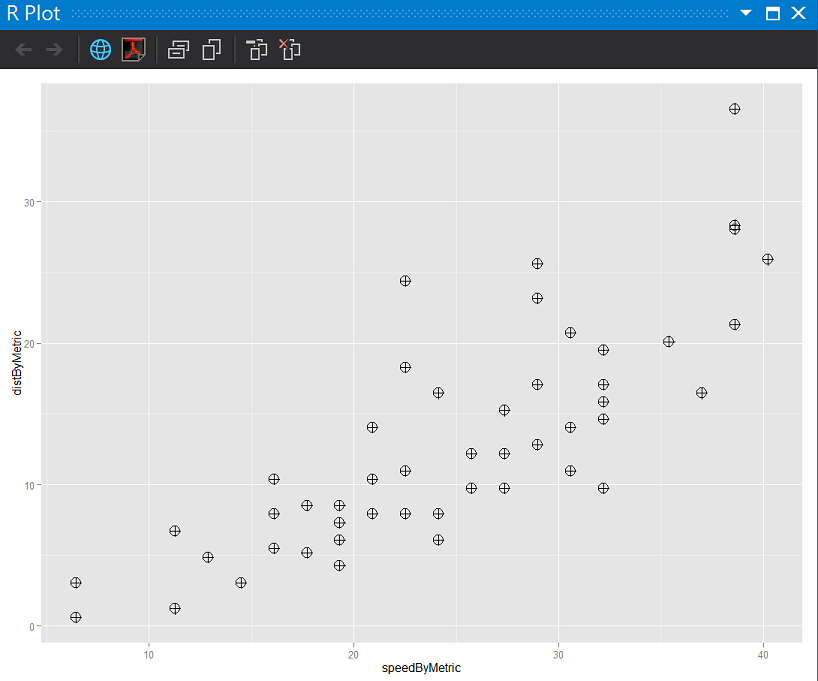2015攝於熊本城，日本九州

R語言與機器學習見面會30

### 1 則留言

0